Uniform Circular Motion and Gravitation

# Newton’s Universal Law of Gravitation

OpenStaxCollege

### Learning Objectives

• Explain Earth’s gravitational force.
• Describe the gravitational effect of the Moon on Earth.
• Discuss weightlessness in space.
• Examine the Cavendish experiment

What do aching feet, a falling apple, and the orbit of the Moon have in common? Each is caused by the gravitational force. Our feet are strained by supporting our weight—the force of Earth’s gravity on us. An apple falls from a tree because of the same force acting a few meters above Earth’s surface. And the Moon orbits Earth because gravity is able to supply the necessary centripetal force at a distance of hundreds of millions of meters. In fact, the same force causes planets to orbit the Sun, stars to orbit the center of the galaxy, and galaxies to cluster together. Gravity is another example of underlying simplicity in nature. It is the weakest of the four basic forces found in nature, and in some ways the least understood. It is a force that acts at a distance, without physical contact, and is expressed by a formula that is valid everywhere in the universe, for masses and distances that vary from the tiny to the immense.

Sir Isaac Newton was the first scientist to precisely define the gravitational force, and to show that it could explain both falling bodies and astronomical motions. See [link]. But Newton was not the first to suspect that the same force caused both our weight and the motion of planets. His forerunner Galileo Galilei had contended that falling bodies and planetary motions had the same cause. Some of Newton’s contemporaries, such as Robert Hooke, Christopher Wren, and Edmund Halley, had also made some progress toward understanding gravitation. But Newton was the first to propose an exact mathematical form and to use that form to show that the motion of heavenly bodies should be conic sections—circles, ellipses, parabolas, and hyperbolas. This theoretical prediction was a major triumph—it had been known for some time that moons, planets, and comets follow such paths, but no one had been able to propose a mechanism that caused them to follow these paths and not others.

According to early accounts, Newton was inspired to make the connection between falling bodies and astronomical motions when he saw an apple fall from a tree and realized that if the gravitational force could extend above the ground to a tree, it might also reach the Sun. The inspiration of Newton’s apple is a part of worldwide folklore and may even be based in fact. Great importance is attached to it because Newton’s universal law of gravitation and his laws of motion answered very old questions about nature and gave tremendous support to the notion of underlying simplicity and unity in nature. Scientists still expect underlying simplicity to emerge from their ongoing inquiries into nature.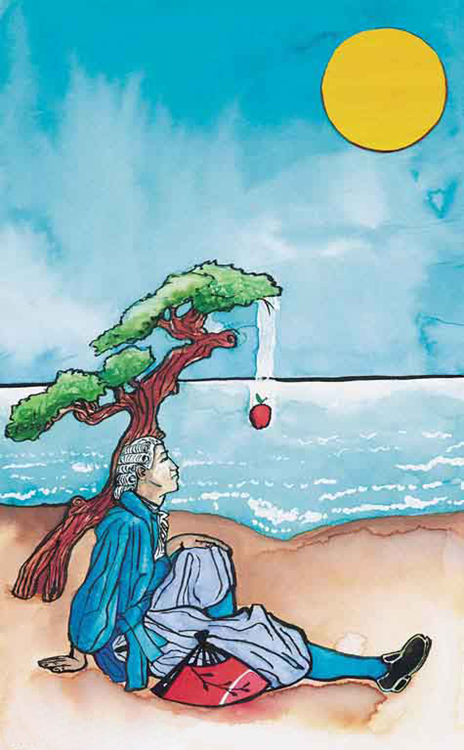The gravitational force is relatively simple. It is always attractive, and it depends only on the masses involved and the distance between them. Stated in modern language, Newton’s universal law of gravitation states that every particle in the universe attracts every other particle with a force along a line joining them. The force is directly proportional to the product of their masses and inversely proportional to the square of the distance between them.

Gravitational attraction is along a line joining the centers of mass of these two bodies. The magnitude of the force is the same on each, consistent with Newton’s third law.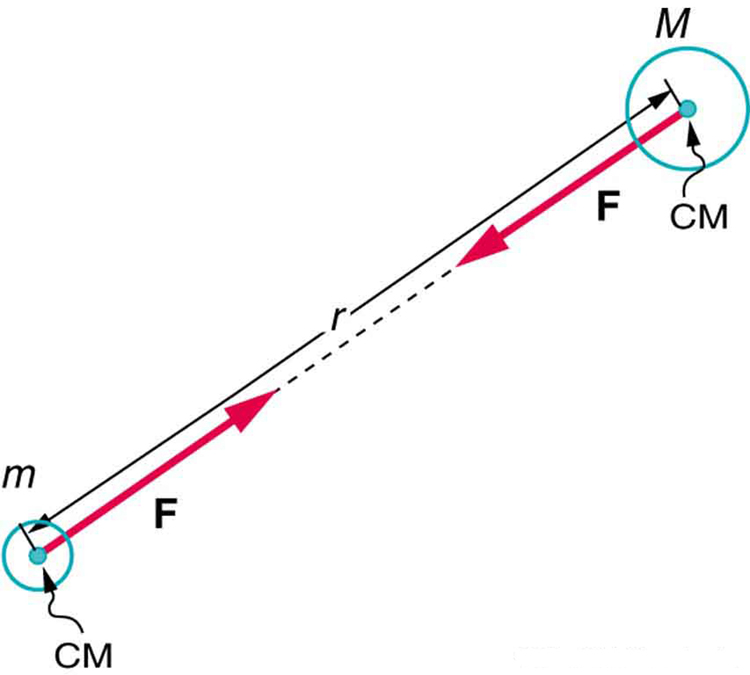The magnitude of the force on each object (one has larger mass than the other) is the same, consistent with Newton’s third law.

The bodies we are dealing with tend to be large. To simplify the situation we assume that the body acts as if its entire mass is concentrated at one specific point called the center of mass (CM), which will be further explored in Linear Momentum and Collisions. For two bodies having masses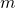and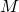with a distance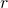between their centers of mass, the equation for Newton’s universal law of gravitation is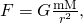where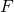is the magnitude of the gravitational force and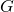is a proportionality factor called the gravitational constant.is a universal gravitational constant—that is, it is thought to be the same everywhere in the universe. It has been measured experimentally to be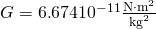in SI units. Note that the units ofare such that a force in newtons is obtained from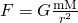, when considering masses in kilograms and distance in meters. For example, two 1.000 kg masses separated by 1.000 m will experience a gravitational attraction of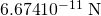. This is an extraordinarily small force. The small magnitude of the gravitational force is consistent with everyday experience. We are unaware that even large objects like mountains exert gravitational forces on us. In fact, our body weight is the force of attraction of the entire Earth on us with a mass of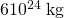.

Recall that the acceleration due to gravity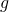is about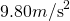on Earth. We can now determine why this is so. The weight of an object mg is the gravitational force between it and Earth. Substituting mg forin Newton’s universal law of gravitation gives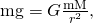whereis the mass of the object,is the mass of Earth, andis the distance to the center of Earth (the distance between the centers of mass of the object and Earth). See [link]. The massof the object cancels, leaving an equation for: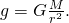Substituting known values for Earth’s mass and radius (to three significant figures),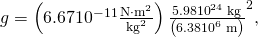and we obtain a value for the acceleration of a falling body: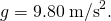The distance between the centers of mass of Earth and an object on its surface is very nearly the same as the radius of Earth, because Earth is so much larger than the object.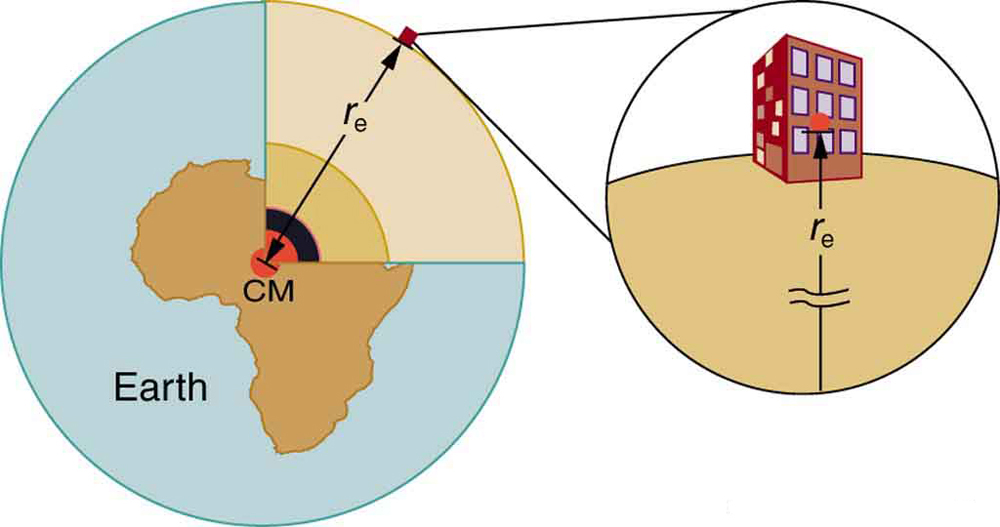This is the expected value and is independent of the body’s mass. Newton’s law of gravitation takes Galileo’s observation that all masses fall with the same acceleration a step further, explaining the observation in terms of a force that causes objects to fall—in fact, in terms of a universally existing force of attraction between masses.

Take-Home Experiment

Take a marble, a ball, and a spoon and drop them from the same height. Do they hit the floor at the same time? If you drop a piece of paper as well, does it behave like the other objects? Explain your observations.

Making Connections

Attempts are still being made to understand the gravitational force. As we shall see in Particle Physics, modern physics is exploring the connections of gravity to other forces, space, and time. General relativity alters our view of gravitation, leading us to think of gravitation as bending space and time.

In the following example, we make a comparison similar to one made by Newton himself. He noted that if the gravitational force caused the Moon to orbit Earth, then the acceleration due to gravity should equal the centripetal acceleration of the Moon in its orbit. Newton found that the two accelerations agreed “pretty nearly.”

Earth’s Gravitational Force Is the Centripetal Force Making the Moon Move in a Curved Path

(a) Find the acceleration due to Earth’s gravity at the distance of the Moon.

(b) Calculate the centripetal acceleration needed to keep the Moon in its orbit (assuming a circular orbit about a fixed Earth), and compare it with the value of the acceleration due to Earth’s gravity that you have just found.

Strategy for (a)

This calculation is the same as the one finding the acceleration due to gravity at Earth’s surface, except thatis the distance from the center of Earth to the center of the Moon. The radius of the Moon’s nearly circular orbit is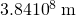.

Solution for (a)

Substituting known values into the expression forfound above, remembering thatis the mass of Earth not the Moon, yields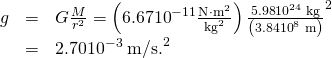Strategy for (b)

Centripetal acceleration can be calculated using either form of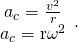We choose to use the second form: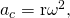where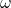is the angular velocity of the Moon about Earth.

Solution for (b)

Given that the period (the time it takes to make one complete rotation) of the Moon’s orbit is 27.3 days, (d) and using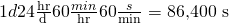we see that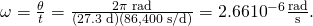The centripetal acceleration is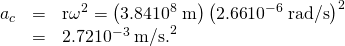The direction of the acceleration is toward the center of the Earth.

Discussion

The centripetal acceleration of the Moon found in (b) differs by less than 1% from the acceleration due to Earth’s gravity found in (a). This agreement is approximate because the Moon’s orbit is slightly elliptical, and Earth is not stationary (rather the Earth-Moon system rotates about its center of mass, which is located some 1700 km below Earth’s surface). The clear implication is that Earth’s gravitational force causes the Moon to orbit Earth.

Why does Earth not remain stationary as the Moon orbits it? This is because, as expected from Newton’s third law, if Earth exerts a force on the Moon, then the Moon should exert an equal and opposite force on Earth (see [link]). We do not sense the Moon’s effect on Earth’s motion, because the Moon’s gravity moves our bodies right along with Earth but there are other signs on Earth that clearly show the effect of the Moon’s gravitational force as discussed in Satellites and Kepler’s Laws: An Argument for Simplicity.

(a) Earth and the Moon rotate approximately once a month around their common center of mass. (b) Their center of mass orbits the Sun in an elliptical orbit, but Earth’s path around the Sun has “wiggles” in it. Similar wiggles in the paths of stars have been observed and are considered direct evidence of planets orbiting those stars. This is important because the planets’ reflected light is often too dim to be observed.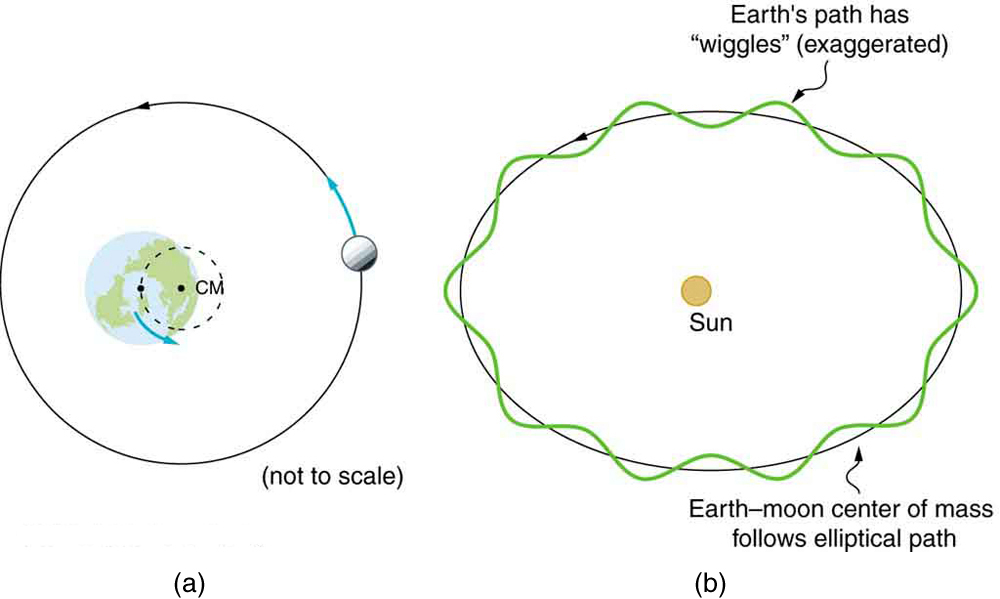# Tides

Ocean tides are one very observable result of the Moon’s gravity acting on Earth. [link] is a simplified drawing of the Moon’s position relative to the tides. Because water easily flows on Earth’s surface, a high tide is created on the side of Earth nearest to the Moon, where the Moon’s gravitational pull is strongest. Why is there also a high tide on the opposite side of Earth? The answer is that Earth is pulled toward the Moon more than the water on the far side, because Earth is closer to the Moon. So the water on the side of Earth closest to the Moon is pulled away from Earth, and Earth is pulled away from water on the far side. As Earth rotates, the tidal bulge (an effect of the tidal forces between an orbiting natural satellite and the primary planet that it orbits) keeps its orientation with the Moon. Thus there are two tides per day (the actual tidal period is about 12 hours and 25.2 minutes), because the Moon moves in its orbit each day as well).

The Moon causes ocean tides by attracting the water on the near side more than Earth, and by attracting Earth more than the water on the far side. The distances and sizes are not to scale. For this simplified representation of the Earth-Moon system, there are two high and two low tides per day at any location, because Earth rotates under the tidal bulge.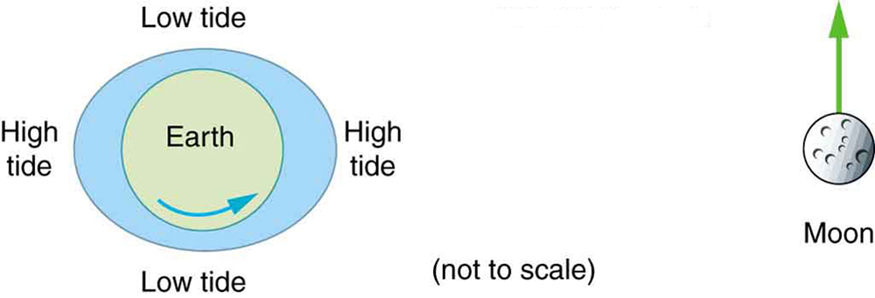The Sun also affects tides, although it has about half the effect of the Moon. However, the largest tides, called spring tides, occur when Earth, the Moon, and the Sun are aligned. The smallest tides, called neap tides, occur when the Sun is at a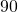angle to the Earth-Moon alignment.

(a, b) Spring tides: The highest tides occur when Earth, the Moon, and the Sun are aligned. (c) Neap tide: The lowest tides occur when the Sun lies atto the Earth-Moon alignment. Note that this figure is not drawn to scale.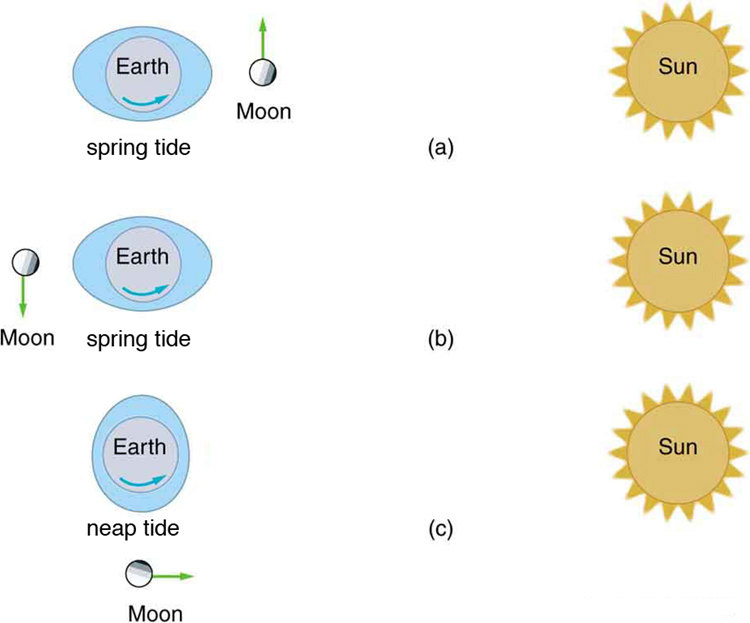Tides are not unique to Earth but occur in many astronomical systems. The most extreme tides occur where the gravitational force is the strongest and varies most rapidly, such as near black holes (see [link]). A few likely candidates for black holes have been observed in our galaxy. These have masses greater than the Sun but have diameters only a few kilometers across. The tidal forces near them are so great that they can actually tear matter from a companion star.

A black hole is an object with such strong gravity that not even light can escape it. This black hole was created by the supernova of one star in a two-star system. The tidal forces created by the black hole are so great that it tears matter from the companion star. This matter is compressed and heated as it is sucked into the black hole, creating light and X-rays observable from Earth.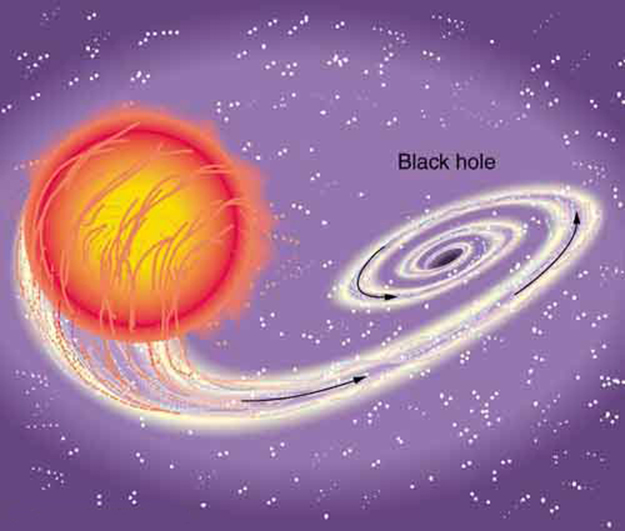# ”Weightlessness” and Microgravity

In contrast to the tremendous gravitational force near black holes is the apparent gravitational field experienced by astronauts orbiting Earth. What is the effect of “weightlessness” upon an astronaut who is in orbit for months? Or what about the effect of weightlessness upon plant growth? Weightlessness doesn’t mean that an astronaut is not being acted upon by the gravitational force. There is no “zero gravity” in an astronaut’s orbit. The term just means that the astronaut is in free-fall, accelerating with the acceleration due to gravity. If an elevator cable breaks, the passengers inside will be in free fall and will experience weightlessness. You can experience short periods of weightlessness in some rides in amusement parks.

Astronauts experiencing weightlessness on board the International Space Station. (credit: NASA)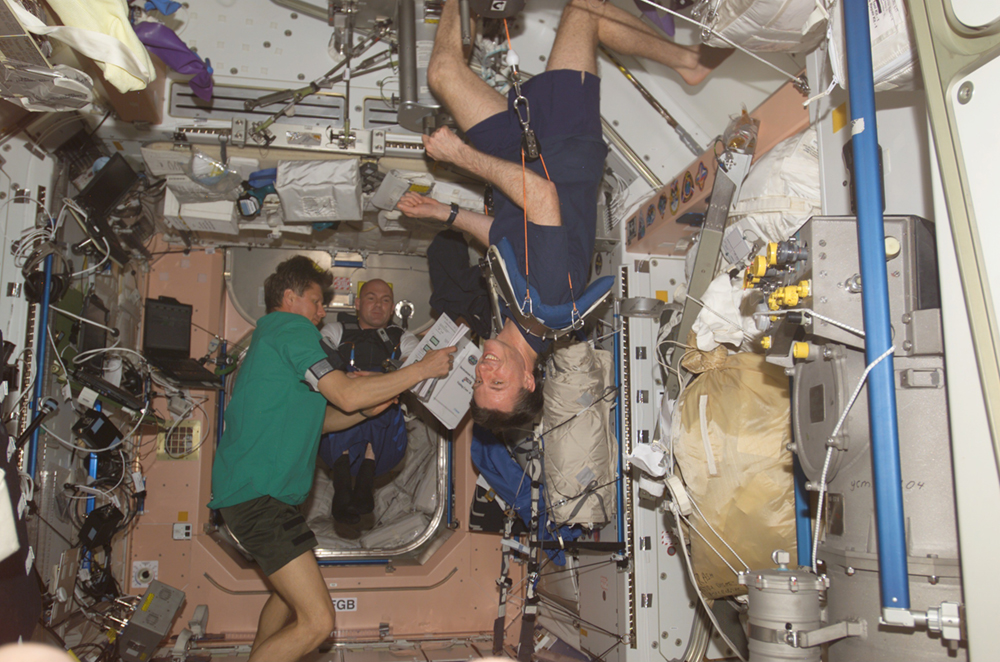Microgravity refers to an environment in which the apparent net acceleration of a body is small compared with that produced by Earth at its surface. Many interesting biology and physics topics have been studied over the past three decades in the presence of microgravity. Of immediate concern is the effect on astronauts of extended times in outer space, such as at the International Space Station. Researchers have observed that muscles will atrophy (waste away) in this environment. There is also a corresponding loss of bone mass. Study continues on cardiovascular adaptation to space flight. On Earth, blood pressure is usually higher in the feet than in the head, because the higher column of blood exerts a downward force on it, due to gravity. When standing, 70% of your blood is below the level of the heart, while in a horizontal position, just the opposite occurs. What difference does the absence of this pressure differential have upon the heart?

Some findings in human physiology in space can be clinically important to the management of diseases back on Earth. On a somewhat negative note, spaceflight is known to affect the human immune system, possibly making the crew members more vulnerable to infectious diseases. Experiments flown in space also have shown that some bacteria grow faster in microgravity than they do on Earth. However, on a positive note, studies indicate that microbial antibiotic production can increase by a factor of two in space-grown cultures. One hopes to be able to understand these mechanisms so that similar successes can be achieved on the ground. In another area of physics space research, inorganic crystals and protein crystals have been grown in outer space that have much higher quality than any grown on Earth, so crystallography studies on their structure can yield much better results.

Plants have evolved with the stimulus of gravity and with gravity sensors. Roots grow downward and shoots grow upward. Plants might be able to provide a life support system for long duration space missions by regenerating the atmosphere, purifying water, and producing food. Some studies have indicated that plant growth and development are not affected by gravity, but there is still uncertainty about structural changes in plants grown in a microgravity environment.

# The Cavendish Experiment: Then and Now

As previously noted, the universal gravitational constantis determined experimentally. This definition was first done accurately by Henry Cavendish (1731–1810), an English scientist, in 1798, more than 100 years after Newton published his universal law of gravitation. The measurement ofis very basic and important because it determines the strength of one of the four forces in nature. Cavendish’s experiment was very difficult because he measured the tiny gravitational attraction between two ordinary-sized masses (tens of kilograms at most), using apparatus like that in [link]. Remarkably, his value fordiffers by less than 1% from the best modern value.

One important consequence of knowingwas that an accurate value for Earth’s mass could finally be obtained. This was done by measuring the acceleration due to gravity as accurately as possible and then calculating the mass of Earthfrom the relationship Newton’s universal law of gravitation giveswhereis the mass of the object,is the mass of Earth, andis the distance to the center of Earth (the distance between the centers of mass of the object and Earth). See [link]. The massof the object cancels, leaving an equation for:Rearranging to solve foryieldsSocan be calculated because all quantities on the right, including the radius of Earth, are known from direct measurements. We shall see in Satellites and Kepler’s Laws: An Argument for Simplicity that knowingalso allows for the determination of astronomical masses. Interestingly, of all the fundamental constants in physics,is by far the least well determined.

The Cavendish experiment is also used to explore other aspects of gravity. One of the most interesting questions is whether the gravitational force depends on substance as well as mass—for example, whether one kilogram of lead exerts the same gravitational pull as one kilogram of water. A Hungarian scientist named Roland von Eötvös pioneered this inquiry early in the 20th century. He found, with an accuracy of five parts per billion, that the gravitational force does not depend on the substance. Such experiments continue today, and have improved upon Eötvös’ measurements. Cavendish-type experiments such as those of Eric Adelberger and others at the University of Washington, have also put severe limits on the possibility of a fifth force and have verified a major prediction of general relativity—that gravitational energy contributes to rest mass. Ongoing measurements there use a torsion balance and a parallel plate (not spheres, as Cavendish used) to examine how Newton’s law of gravitation works over sub-millimeter distances. On this small-scale, do gravitational effects depart from the inverse square law? So far, no deviation has been observed.

Cavendish used an apparatus like this to measure the gravitational attraction between the two suspended spheres () and the two on the stand () by observing the amount of torsion (twisting) created in the fiber. Distance between the masses can be varied to check the dependence of the force on distance. Modern experiments of this type continue to explore gravity.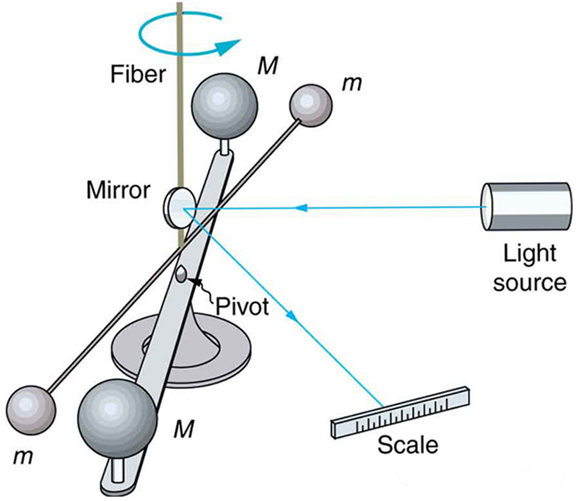# Section Summary

• Newton’s universal law of gravitation: Every particle in the universe attracts every other particle with a force along a line joining them. The force is directly proportional to the product of their masses and inversely proportional to the square of the distance between them. In equation form, this iswhere F is the magnitude of the gravitational force.is the gravitational constant, given by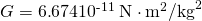.

• Newton’s law of gravitation applies universally.

# Conceptual Questions

Action at a distance, such as is the case for gravity, was once thought to be illogical and therefore untrue. What is the ultimate determinant of the truth in physics, and why was this action ultimately accepted?

Two friends are having a conversation. Anna says a satellite in orbit is in freefall because the satellite keeps falling toward Earth. Tom says a satellite in orbit is not in freefall because the acceleration due to gravity is not. Who do you agree with and why?

Draw a free body diagram for a satellite in an elliptical orbit showing why its speed increases as it approaches its parent body and decreases as it moves away.

Newton’s laws of motion and gravity were among the first to convincingly demonstrate the underlying simplicity and unity in nature. Many other examples have since been discovered, and we now expect to find such underlying order in complex situations. Is there proof that such order will always be found in new explorations?

# Problem Exercises

(a) Calculate Earth’s mass given the acceleration due to gravity at the North Pole is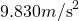and the radius of the Earth is 6371 km from center to pole.

(b) Compare this with the accepted value of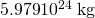.

a)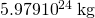b) This is identical to the best value to three significant figures.

(a) Calculate the magnitude of the acceleration due to gravity on the surface of Earth due to the Moon.

(b) Calculate the magnitude of the acceleration due to gravity at Earth due to the Sun.

(c) Take the ratio of the Moon’s acceleration to the Sun’s and comment on why the tides are predominantly due to the Moon in spite of this number.

(a) What is the acceleration due to gravity on the surface of the Moon?

(b) On the surface of Mars? The mass of Mars is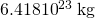and its radius is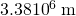.

a)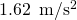b)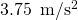(a) Calculate the acceleration due to gravity on the surface of the Sun.

(b) By what factor would your weight increase if you could stand on the Sun? (Never mind that you cannot.)

The Moon and Earth rotate about their common center of mass, which is located about 4700 km from the center of Earth. (This is 1690 km below the surface.)

(a) Calculate the magnitude of the acceleration due to the Moon’s gravity at that point.

(b) Calculate the magnitude of the centripetal acceleration of the center of Earth as it rotates about that point once each lunar month (about 27.3 d) and compare it with the acceleration found in part (a). Comment on whether or not they are equal and why they should or should not be.

a)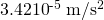b)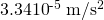The values are nearly identical. One would expect the gravitational force to be the same as the centripetal force at the core of the system.

Solve part (b) of [link] using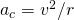.

Astrology, that unlikely and vague pseudoscience, makes much of the position of the planets at the moment of one’s birth. The only known force a planet exerts on Earth is gravitational.

(a) Calculate the magnitude of the gravitational force exerted on a 4.20 kg baby by a 100 kg father 0.200 m away at birth (he is assisting, so he is close to the child).

(b) Calculate the magnitude of the force on the baby due to Jupiter if it is at its closest distance to Earth, some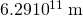away. How does the force of Jupiter on the baby compare to the force of the father on the baby? Other objects in the room and the hospital building also exert similar gravitational forces. (Of course, there could be an unknown force acting, but scientists first need to be convinced that there is even an effect, much less that an unknown force causes it.)

a)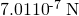b)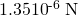,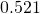The existence of the dwarf planet Pluto was proposed based on irregularities in Neptune’s orbit. Pluto was subsequently discovered near its predicted position. But it now appears that the discovery was fortuitous, because Pluto is small and the irregularities in Neptune’s orbit were not well known. To illustrate that Pluto has a minor effect on the orbit of Neptune compared with the closest planet to Neptune:

(a) Calculate the acceleration due to gravity at Neptune due to Pluto when they are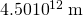apart, as they are at present. The mass of Pluto is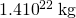.

(b) Calculate the acceleration due to gravity at Neptune due to Uranus, presently about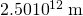apart, and compare it with that due to Pluto. The mass of Uranus is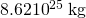.

(a) The Sun orbits the Milky Way galaxy once each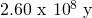, with a roughly circular orbit averaging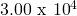light years in radius. (A light year is the distance traveled by light in 1 y.) Calculate the centripetal acceleration of the Sun in its galactic orbit. Does your result support the contention that a nearly inertial frame of reference can be located at the Sun?

(b) Calculate the average speed of the Sun in its galactic orbit. Does the answer surprise you?

a)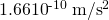b)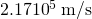Unreasonable Result

A mountain 10.0 km from a person exerts a gravitational force on him equal to 2.00% of his weight.

(a) Calculate the mass of the mountain.

(b) Compare the mountain’s mass with that of Earth.

(c) What is unreasonable about these results?

(d) Which premises are unreasonable or inconsistent? (Note that accurate gravitational measurements can easily detect the effect of nearby mountains and variations in local geology.)

a)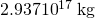b)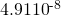of the Earth’s mass.

c) The mass of the mountain and its fraction of the Earth’s mass are too great.

d) The gravitational force assumed to be exerted by the mountain is too great.

## Glossary

gravitational constant, G
a proportionality factor used in the equation for Newton’s universal law of gravitation; it is a universal constant—that is, it is thought to be the same everywhere in the universe
center of mass
the point where the entire mass of an object can be thought to be concentrated
microgravity
an environment in which the apparent net acceleration of a body is small compared with that produced by Earth at its surface
Newton’s universal law of gravitation
every particle in the universe attracts every other particle with a force along a line joining them; the force is directly proportional to the product of their masses and inversely proportional to the square of the distance between them